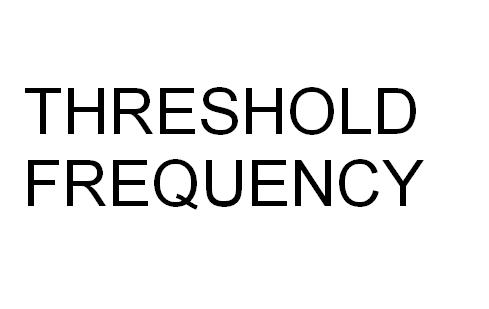# Difference Between Threshold Frequency and Work Function

Work function and threshold frequency are related to the photoelectric effect. This effect is widely used in the experimentation that demonstrates nature of waves. There are two basic differences between threshold frequency and work function.

While threshold frequency’s unit of measurement is hertz, work function can be measured in either joules or electron volts.

Secondly, in order to apply threshold frequency, the frequency itself must be multiplied by the plank constant so the corresponding energy can be obtained. On the other hand, a work function can simply be applied to the equation of photoelectric effect given by Albert Einstein.

### Instructions

• 1

Threshold Frequency

In order to fully understand the concept of threshold frequency, you need to know the idea behind the photoelectric effect. Now, what is the photoelectric effect?

In case of an incidental electromagnetic radiation, the process in which there is an ejection of an electron from a metal, it is known as the photoelectric effect.

There is always a threshold frequency for the waves which are caused incidentally. No matter what the intensity of the electromagnetic waves is, the electrons will not get ejected unless the criterion of the required frequency is matched. The time difference between the time of incidence of light and the ejection of electrons is almost a thousandth value which is derived from the wave theory.

Once the light is produced using threshold frequency, the electrons ejected depend upon the intensity at which the light is travelling. The kinetic energy of the ejected electrons is dependent upon the frequency of the same incident light.

This leads to the photon theory, which means that when light interacts with matter, it behaves as particles. This light travelling in particles comes in the form of small energy packets, photons. The energy of each photon is directly dependent upon its frequency. The formula E = h f (E: energy, h: plank constant, f: frequency) helps you calculate the frequency and energy of photon.

This observation clearly showcases how the electrons absorb photons only when one photon has the energy to take an electron to a stable state. This is threshold frequency and it is denoted by the term ft.• 2

Work Function

Once the energy corresponds with the threshold frequency of a metal, it is the metal’s work function. The Greek letter φ is used to denote this scientific phenomenon. Einstein claimed that the kinetic energy of an ejected electron was directly dependent on the frequency of the photon and its work function. The formula K.E. (max) = h f – φ explains this concept perfectly. Here K.E. means the Kinetic Energy; h is the plank constant while f is the incident frequency.

The formula estimates how the kinetic energy of the ejected electron can be zero if the energy from the incident photon is equal to its work function (φ).•